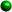Homework Tips for the WeekFactoring Trinomials The signs are key. Look at the sign of the third term.If it is positive, the signs in your answer will be the same, either both plus or both minus depending on the middle term.If it is negative, the signs in your answer will be different. If there is NO coefficient in the first term, it is an easier one where by listing the factors of the third term we can see which factors add or subtract to give us the middle term. Always check by FOIL!If there is a coefficient in the first term, the problem is a little harder and we must factor by trial and error. Don't get frustrated.It is factoring by trial and error. Use a pencil with an eraser! Sometimes you can get so involved with the numbers, you forget to check the signs, be careful. For examples and further explanation especially the trinomials with a coefficient in the first term see: Student Tutorials- Factoring Trinomials